# 【算法】五分钟算法小知识：动态规划之博弈问题-问答-阿里云开发者社区-阿里云

## 【算法】五分钟算法小知识：动态规划之博弈问题游客ih62co2qqq5ww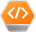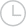2020-05-14 09:56:12565

### 一、定义 dp 数组的含义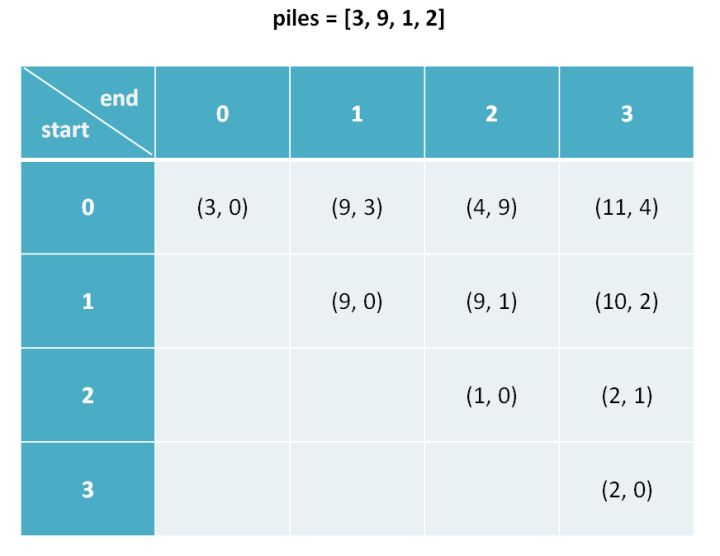``````dp[i][j].fir 表示，对于 piles[i...j] 这部分石头堆，先手能获得的最高分数。
dp[i][j].sec 表示，对于 piles[i...j] 这部分石头堆，后手能获得的最高分数。

dp.fir = 9 意味着：面对石头堆 [3, 9]，先手最终能够获得 9 分。
dp.sec = 2 意味着：面对石头堆 [9, 1, 2]，后手最终能够获得 2 分。
``````

### 二、状态转移方程

``````dp[i][j][fir or sec]

0 <= i < piles.length
i <= j < piles.length
``````

``````n = piles.length
for 0 <= i < n:
for j <= i < n:
for who in {fir, sec}:
dp[i][j][who] = max(left, right)
``````

``````dp[i][j].fir = max(piles[i] + dp[i+1][j].sec, piles[j] + dp[i][j-1].sec)
dp[i][j].fir = max(    选择最左边的石头堆     ,     选择最右边的石头堆     )
# 解释：我作为先手，面对 piles[i...j] 时，有两种选择：
# 要么我选择最左边的那一堆石头，然后面对 piles[i+1...j]
# 但是此时轮到对方，相当于我变成了后手；
# 要么我选择最右边的那一堆石头，然后面对 piles[i...j-1]
# 但是此时轮到对方，相当于我变成了后手。

if 先手选择左边:
dp[i][j].sec = dp[i+1][j].fir
if 先手选择右边:
dp[i][j].sec = dp[i][j-1].fir
# 解释：我作为后手，要等先手先选择，有两种情况：
# 如果先手选择了最左边那堆，给我剩下了 piles[i+1...j]
# 此时轮到我，我变成了先手；
# 如果先手选择了最右边那堆，给我剩下了 piles[i...j-1]
# 此时轮到我，我变成了先手。
``````

``````dp[i][j].fir = piles[i]
dp[i][j].sec = 0

# 解释：i 和 j 相等就是说面前只有一堆石头 piles[i]
# 那么显然先手的得分为 piles[i]
# 后手没有石头拿了，得分为 0
``````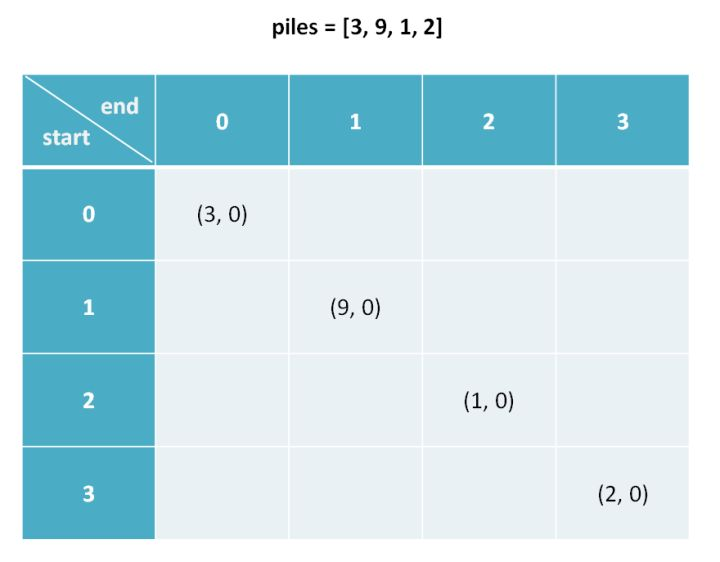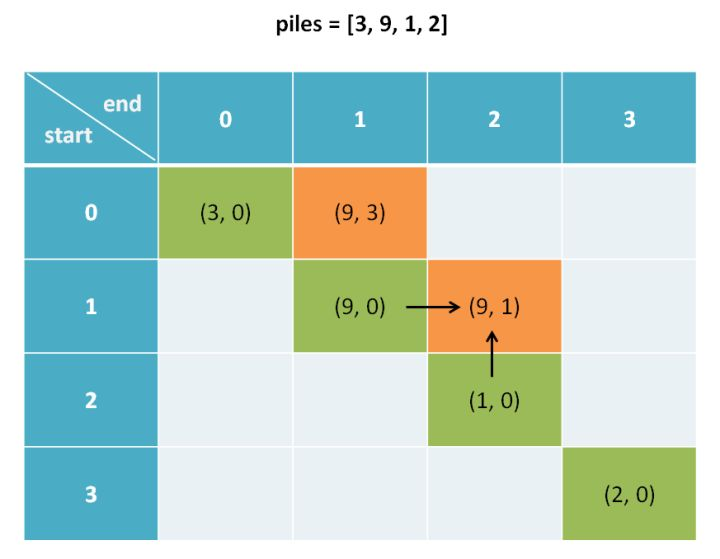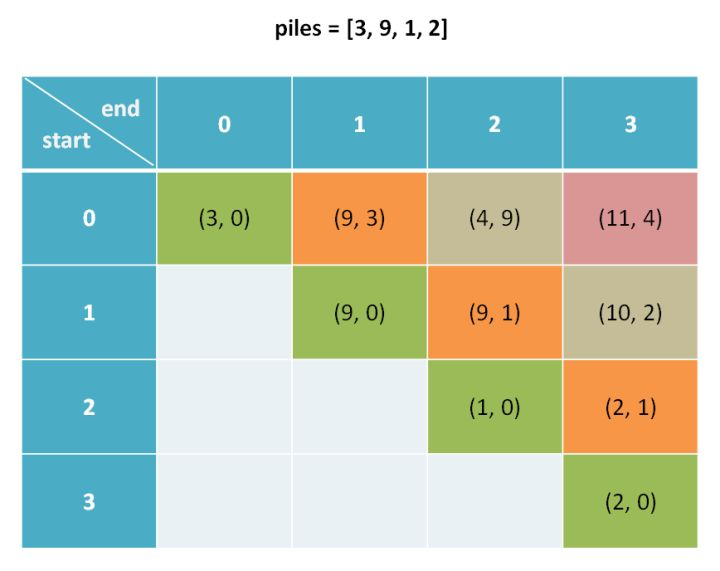### 三、代码实现

``````class Pair {
int fir, sec;
Pair(int fir, int sec) {
this.fir = fir;
this.sec = sec;
}
}
``````

``````/* 返回游戏最后先手和后手的得分之差 */
int stoneGame(int[] piles) {
int n = piles.length;
// 初始化 dp 数组
Pair[][] dp = new Pair[n][n];
for (int i = 0; i < n; i++)
for (int j = i; j < n; j++)
dp[i][j] = new Pair(0, 0);
// 填入 base case
for (int i = 0; i < n; i++) {
dp[i][i].fir = piles[i];
dp[i][i].sec = 0;
}
// 斜着遍历数组
for (int l = 2; l <= n; l++) {
for (int i = 0; i <= n - l; i++) {
int j = l + i - 1;
// 先手选择最左边或最右边的分数
int left = piles[i] + dp[i+1][j].sec;
int right = piles[j] + dp[i][j-1].sec;
// 套用状态转移方程
if (left > right) {
dp[i][j].fir = left;
dp[i][j].sec = dp[i+1][j].fir;
} else {
dp[i][j].fir = right;
dp[i][j].sec = dp[i][j-1].fir;
}
}
}
Pair res = dp[n-1];
return res.fir - res.sec;
}
``````

### 四、最后总结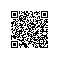取消 提交回答使用钉钉扫一扫加入圈子
+ 订阅